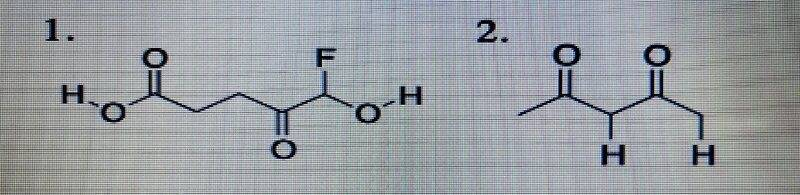# Factors that determine acid strength

• Chemistry
Homework Statement:
In these two compounds, the question is to decide more acidic hydrogen.

In No.1, I think the right hydrogen is more acidic because halogen (Fluorine) connects the oxygen next to the hydrogen, pulls the electron and this inductive effect makes the hydrogen more stable.
But the answer is the left hydrogen.

In No.2, I also think right hydrogen is more acidic because there is a methyl group on the left side of carbon which is electron donating group and makes the left hydrogen unstable.
But the answer is the left hydrogen.
I think there are the mesomeric effect on both of the hydrogens.
Could anyone explain me the reason?
Relevant Equations:
I think to solve this kind of more acidic hydrogen, there are these rules below.
1. Compare the element to which a hydrogen bonds.
2. Check if there is the Resonance effect.
3. Check if there is the Inductive effect.
4. The percentage of the S orbitals. SP>SP2>SP3•